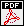## On the non-commutative Main Conjecture for elliptic curves with complex multiplication

Autors: Thanasis Bouganis and Otmar Venjakob
Titel:  On the non-commutative Main Conjecture for elliptic curves with complex multiplication
Jahr: 2010
Seiten: 31
In: Asian J. Math. 14 (2010), no. 3, 385--416.Abstract: In [CFKSV] a non-commutative Iwasawa Main Conjecture for elliptic curves over \$\mathbb{Q}\$ has been formulated. In this note we show that it holds for all CM-elliptic curves \$E\$ defined over \$\mathbb{Q}\$. This was claimed in (loc.\ cit.) without proof, which we want to provide now assuming that the torsion conjecture holds in this case. Based on this we show firstly the existence of the (non-commutative) \$p\$-adic \$L\$-function of \$E\$ and secondly that the (non-commutative) Main Conjecture follows from the existence of the Katz-measure, the work of Yager and Rubin's proof of the 2-variable main conjecture. The main issues are the comparison of the involved periods and to show that the (non-commutative) \$p\$-adic \$L\$-function is defined over the conjectured in (loc.\ cit.) coefficient ring. Moreover we generalize our considerations to the case of CM-elliptic cusp forms. MSC-class: 11R23 (Primary) 11G40 (Secondary)